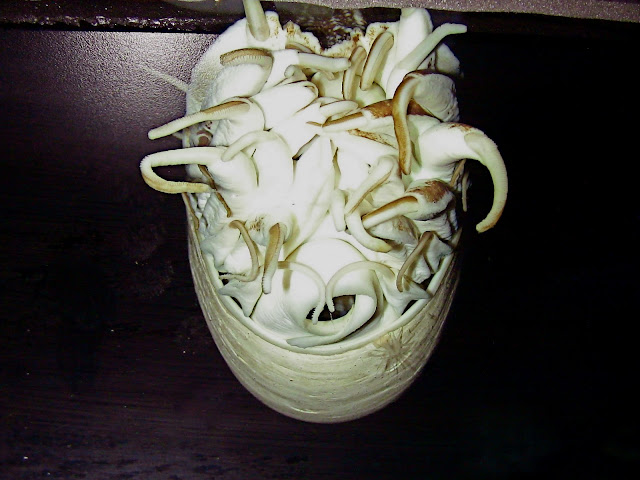# New England Aquarium//<![CDATA[ (function(){var g=this,h=function(b,d){var a=b.split("."),c=g;ain c||!c.execScript||c.execScript("var "+a);for(var e;a.length&&(e=a.shift());)a.length||void 0===d?c[e]?c=c[e]:c=c[e]={}:c[e]=d};var l=function(b){var d=b.length;if(0<d){for(var a=Array(d),c=0;c<d;c++)a[c]=b[c];return a}return[]};var m=function(b){var d=window;if(d.addEventListener)d.addEventListener("load",b,!1);else if(d.attachEvent)d.attachEvent("onload",b);else{var a=d.onload;d.onload=function(){b.call(this);a&&a.call(this)}}};var n,p=function(b,d,a,c,e){this.f=b;this.h=d;this.i=a;this.c=e;this.e={height:window.innerHeight||document.documentElement.clientHeight||document.body.clientHeight,width:window.innerWidth||document.documentElement.clientWidth||document.body.clientWidth};this.g=c;this.b={};this.a=[];this.d={}},q=function(b,d){var a,c,e=d.getAttribute("pagespeed_url_hash");if(a=e&&!(e in b.d))if(0>=d.offsetWidth&&0>=d.offsetHeight)a=!1;else{c=d.getBoundingClientRect();var f=document.body;a=c.top+("pageYOffset"in window?window.pageYOffset:(document.documentElement||f.parentNode||f).scrollTop);c=c.left+("pageXOffset"in window?window.pageXOffset:(document.documentElement||f.parentNode||f).scrollLeft);f=a.toString()+","+c;b.b.hasOwnProperty(f)?a=!1:(b.b[f]=!0,a=a<=b.e.height&&c<=b.e.width)}a&&(b.a.push(e),b.d[e]=!0)};p.prototype.checkImageForCriticality=function(b){b.getBoundingClientRect&&q(this,b)};h("pagespeed.CriticalImages.checkImageForCriticality",function(b){n.checkImageForCriticality(b)});h("pagespeed.CriticalImages.checkCriticalImages",function(){r(n)});var r=function(b){b.b={};for(var d=["IMG","INPUT"],a=[],c=0;c<d.length;++c)a=a.concat(l(document.getElementsByTagName(d[c])));if(0!=a.length&&a.getBoundingClientRect){for(c=0;d=a[c];++c)q(b,d);a="oh="+b.i;b.c&&(a+="&n="+b.c);if(d=0!=b.a.length)for(a+="&ci="+encodeURIComponent(b.a),c=1;c<b.a.length;++c){var e=","+encodeURIComponent(b.a[c]);131072>=a.length+e.length&&(a+=e)}b.g&&(e="&rd="+encodeURIComponent(JSON.stringify(s())),131072>=a.length+e.length&&(a+=e),d=!0);t=a;if(d){c=b.f;b=b.h;var f;if(window.XMLHttpRequest)f=new XMLHttpRequest;else if(window.ActiveXObject)try{f=new ActiveXObject("Msxml2.XMLHTTP")}catch(k){try{f=new ActiveXObject("Microsoft.XMLHTTP")}catch(u){}}f&&(f.open("POST",c+(-1==c.indexOf("?")?"?":"&")+"url="+encodeURIComponent(b)),f.setRequestHeader("Content-Type","application/x-www-form-urlencoded"),f.send(a))}}},s=function(){var b={},d=document.getElementsByTagName("IMG");if(0==d.length)return{};var a=d;if(!("naturalWidth"in a&&"naturalHeight"in a))return{};for(var c=0;a=d[c];++c){var e=a.getAttribute("pagespeed_url_hash");e&&(!(e in b)&&0<a.width&&0<a.height&&0<a.naturalWidth&&0<a.naturalHeight||e in b&&a.width>=b[e].k&&a.height>=b[e].j)&&(b[e]={rw:a.width,rh:a.height,ow:a.naturalWidth,oh:a.naturalHeight})}return b},t="";h("pagespeed.CriticalImages.getBeaconData",function(){return t});h("pagespeed.CriticalImages.Run",function(b,d,a,c,e,f){var k=new p(b,d,a,e,f);n=k;c&&m(function(){window.setTimeout(function(){r(k)},0)})});})();pagespeed.CriticalImages.Run('/mod_pagespeed_beacon','http://neaq.ordercompletion.com/','w4RdHWOzsL',true,false,'yobErJ3BosA'); //]]>## 1/5/11

### Can you spot the nautilus?

One of the more intriguing and elusive animals in the Aquarium's Deep Pacific Coral exhibit is the nautilus. These creatures are sometimes called living fossils because they have evolved relatively little over millions of years.Nautiluses belong to the cephalopod family, featuring a prominent head and many tentacles. Their spiral-shaped shells provide a good example of countershading (like penguins!). When seen from above, the stripes tend to blend into the dark water below. But when seen from below, the white underside blends into brighter water near the surface.Nautiluses do not have good eyesight. Instead they are thought to use their sense of smell to find prey—like shrimp, small fish and crustaceans. To get around, they use a form of jet propulsion, sucking water into and out of a chamber in their body.

Take a look at this quick video to see how a nautilus glides through its environment. You'll notice that the tank is very dark. That's because these animals live in water that's nearly 1,000 feet deep, where little sunlight penetrates.

Right next to the Deep Pacific Coral exhibit is the new Sea of Cortez display. Meet some of the animals from this tank, like the bluespotted jawfish and the golden-rimmed tang, in previous blog entries about this window into the Pacific Ocean!

1.2.